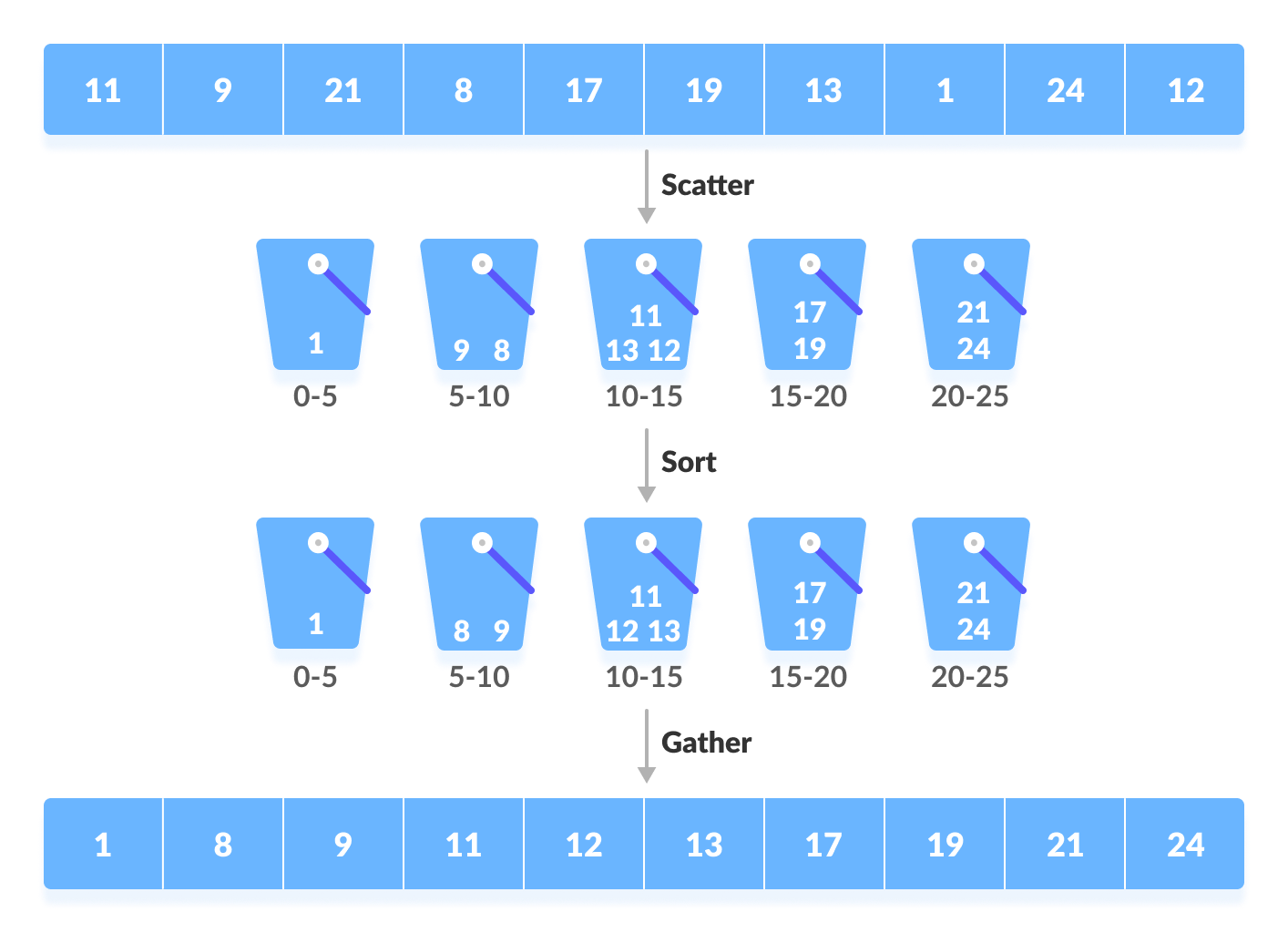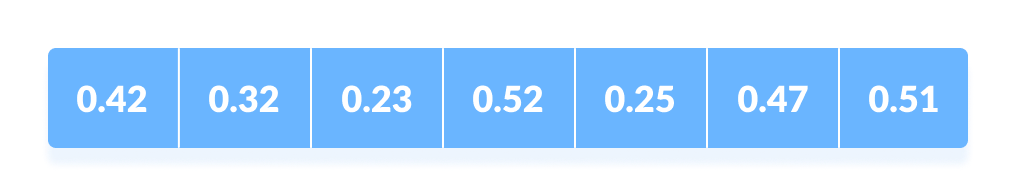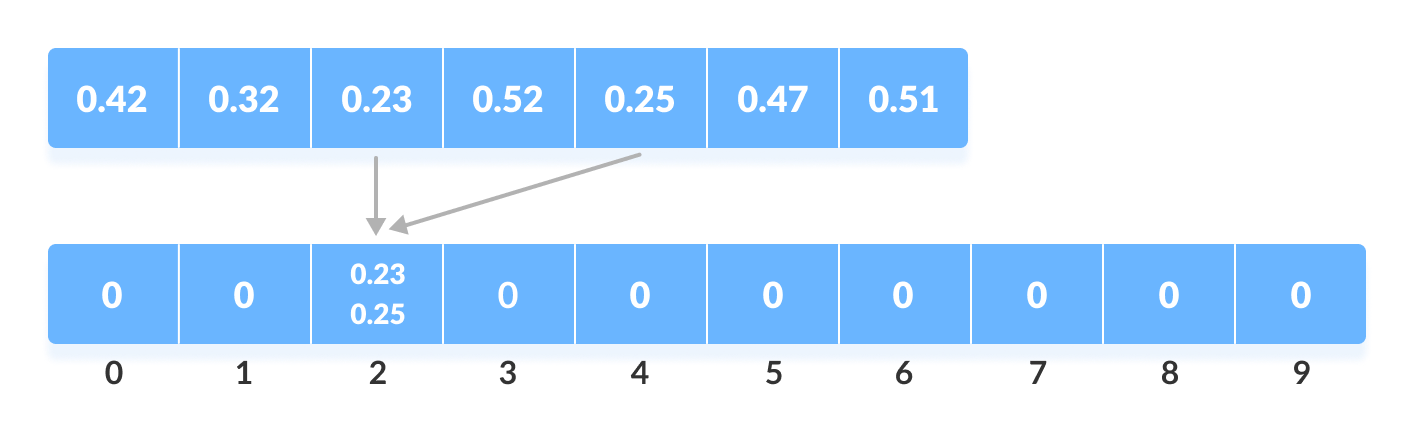# Bucket Sort Algorithm

In this tutorial, you will learn how bucket sort works. Also, you will find working examples of bucket sort in C, C++, Java and Python.

Bucket Sort is a sorting technique that sorts the elements by first dividing the elements into several groups called buckets. The elements inside each bucket are sorted using any of the suitable sorting algorithms or recursively calling the same algorithm.

A number of buckets are created. Each bucket is filled with a specific range of elements. The elements inside the bucket are sorted using any other algorithm. Finally, the elements of the bucket are gathered to get the sorted array.

The process of bucket sort can be understood as scatter-gather approach. The elements are first scattered into buckets then the elements of buckets are sorted. Finally, the elements are gathered in order.## How Bucket Sort Works?

1. Suppose, the input array is:Create an array of size 10. Each slot of this array is used as a bucket for storing elements.2. Insert elements into the buckets from the array. The elements are inserted according to the range of the bucket.

In our example code, we have buckets each of ranges from 0 to 1, 1 to 2, 2 to 3,...... (n-1) to n.
Suppose, an input element is `.23` is taken. It is multiplied by `size = 10` (ie. `.23*10=2.3`). Then, it is converted into an integer (ie. `2.3≈2`). Finally, .23 is inserted into bucket-2.Similarly, .25 is also inserted into the same bucket. Everytime, the floor value of thr floating point number is taken.

In a similar way, other elements are inserted into their respective buckets.3. The elements of each bucket are sorted using any of the stable sorting algorithms. Here, we have used quicksort (inbuilt function).4. The elements from each bucket are gathered.

It is done by iterating through the bucket and inserting an individual element into the original array in each cycle. The element from the bucket is erased once it is copied into the original array.## Bucket Sort Algorithm

``````bucketSort()
create N buckets each of which can hold a range of values
for all the buckets
initialize each bucket with 0 values
for all the buckets
put elements into buckets matching the range
for all the buckets
sort elements in each bucket
gather elements from each bucket
end bucketSort``````

## Python, Java and C/C++ Examples

``````# Bucket Sort in Python programming

def bucketSort(array):
bucket = []

for i in range(len(array)):
bucket.append([])

for j in array:
index_b = int(10 * j)
bucket[index_b].append(j)

for i in range(len(array)):
bucket[i] = sorted(bucket[i])

k = 0
for i in range(len(array)):
for j in range(len(bucket[i])):
array[k] = bucket[i][j]
k += 1
return array

array = [.42, .32, .33, .52, .37, .47, .51]
print("Sorted Array in descending order is")
print(bucketSort(array))``````
``````// Bucket Sort in Java programming

import java.util.*;

class BucketSort {
public static void bucketSort(int[] array, int size) {
int max = (Arrays.stream(array).max().getAsInt());
int[] bucket = new int[max + 1];
for (int i = 0; i <= max; i++) {
bucket[i] = 0;
}

for (int i = 0; i < size; i++) {
bucket[array[i]]++;
}

for (int i = 0, j = 0; i <= max; i++) {
while (bucket[i] > 0) {
array[j++] = i;
bucket[i]--;
}
}
}

public static void main(String[] args) {
int[] data = { 4, 3, 4, 5, 6, 9, 1, 5 };
int size = data.length;
BucketSort bs = new BucketSort();
bs.bucketSort(data, size);
System.out.println("Sorted Array in Ascending Order: " + Arrays.toString(data));
}
}``````
``````// Bucket Sort in C programming

#include <stdio.h>
int getMax(int array[], int size)
{
int max = array;
for (int i = 1; i < size; i++)
if (array[i] > max)
max = array[i];
return max;
}
void bucketSort(int array[], int size)
{
// The size of bucket must be at least the (max+1) but
// we cannot assign declare it as int bucket(max+1) in C as
// it does not support dynamic memory allocation.
// So, its size is provided statically.
int bucket;
const int max = getMax(array, size);
for (int i = 0; i <= max; i++)
{
bucket[i] = 0;
}
for (int i = 0; i < size; i++)
{
bucket[array[i]]++;
}
for (int i = 0, j = 0; i <= max; i++)
{
while (bucket[i] > 0)
{
array[j++] = i;
bucket[i]--;
}
}
}
void printArray(int array[], int size)
{
for (int i = 0; i < size; ++i)
{
printf("%d  ", array[i]);
}
printf("\n");
}
int main()
{
int data[] = {4, 3, 4, 5, 6, 0, 9, 5};
int size = sizeof(data) / sizeof(data);
bucketSort(data, size);
printf("Sorted array in ascending order: \n");
printArray(data, size);
}``````
``````// Bucket Sort in C++ programming

#include <iostream>
#include <vector>
#include <algorithm>
using namespace std;

void bucketSort(float *array, int size)
{
vector<float> bucket[size];

for (int i = 0; i < size; i++)
{
bucket[int(array[i]) * 10].push_back(array[i]);
}
for (int i = 0; i < size; i++)
{
sort(bucket[i].begin(), bucket[i].end());
}
int index = 0;
for (int i = 0; i < size; i++)
{
while (!bucket[i].empty())
{
array[index++] = *(bucket[i].begin());
bucket[i].erase(bucket[i].begin());
}
}
}
void printArray(float *array, int size)
{
for (int i = 0; i < size; i++)
cout << array[i] << " ";
cout << endl;
}
int main()
{
float data[] = {.42, .32, .33, .52, .37, .47, .51};
int size = sizeof(data) / sizeof(data);
bucketSort(data, size);
cout << "Sorted array in ascending order: \n";
printArray(data, size);
}``````

## Complexity

• Worst Case Complexity: `O(n2)`

When there are elements of close range in the array, they are likely to be placed in the same bucket. This may result in some buckets having more number of elements than others.

It makes the complexity depend on the sorting algorithm used to sort the elements of the bucket.

The copmlexity becomes even worse when the elements are in reverse order. If insertion sort is used to sort elements of the bucket, then the time complexity becomes `O(n2)`.

• Best Case Complexity: `O(n+k)`

It occurs when the elements are uniformly distributed in the buckets with a nearly equal number of elements in each bucket.

The complexity becomes even better if the elements inside the buckets are already sorted.

If insertion sort is used to sort elements of a bucket then the overall complexity in the best case will be linear ie. `O(n+k)`. `O(n)` is the complexity for making the buckets and `O(k)` is the complexity for sorting the elements of the bucket using algorithm having linear time complexity at best case.

• Average Case Complexity: `O(n)`

It occurs when the elements are distributed randomly in the array. Even if the elements are not distributed uniformly, bucket sort runs in linear time. It holds true until the sum of the squares of the bucket sizes is linear in the total number of elements.

## Bucket Sort Applications

Bucket sort is used when:

• input is uniformly distributed over a range.
• there are floating point values